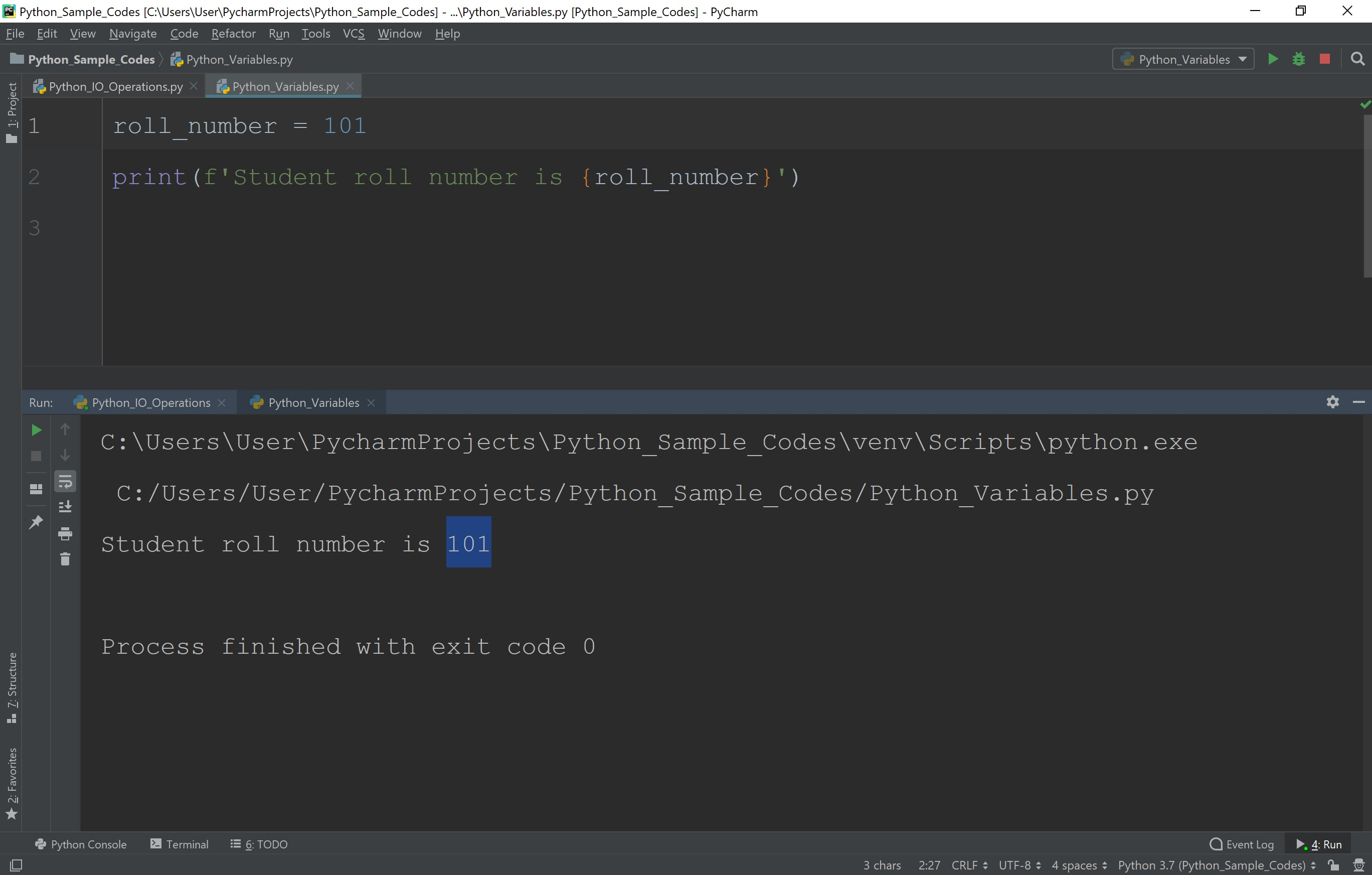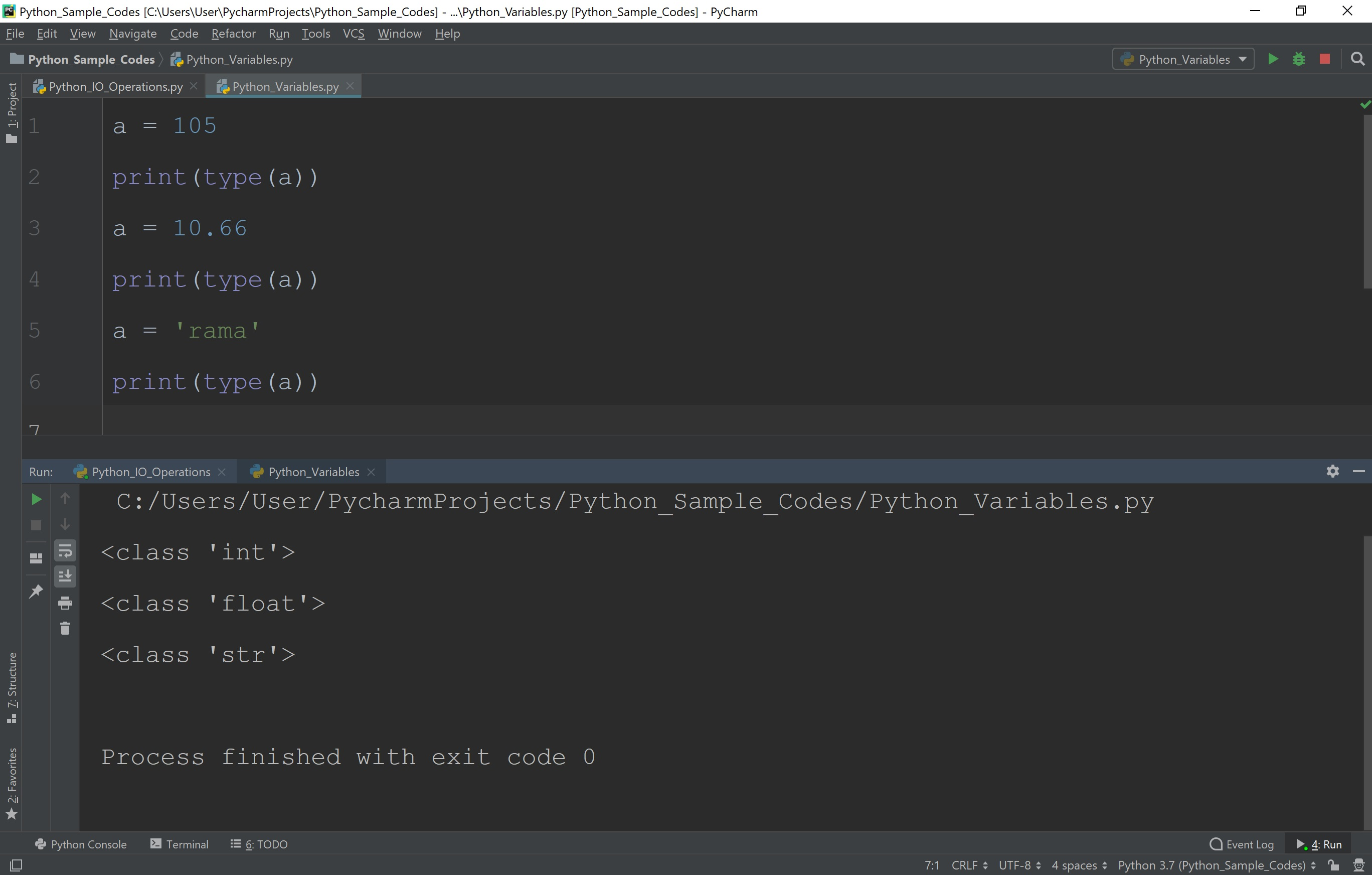The perfect place for easy learning...

×

# Python Variables

In Python, a variable is a named memory where a programmer can store data and retrieve for future use using the same name. In Python, variables are created without specifying any data type. There is no specific keyword used to create a variable. Variables are created directly by specifying the variable name with a value.

We use the following syntax to create a variable.

### Syntax

variable_name = value

When a variable is defined, we must create it with a value. If the value is not assigned the variable gives an error stating that variable is not defined. Consider the following example Python code.

# Python code to illustrate variable declaration error

``````
roll_number
print(f'Student roll number is {roll_number}')
```
```

When we run the above code, it produces an error message as follows.So when a variable has created, we must assign a value to it. Look at the following Python code.

# Python code to illustrate variable declaration

``````
roll_number = 101
print(f'Student roll number is {roll_number}')
```
```

When we run the above code, it produces the output as follows.## Declaring multiple variables in a single statement

In Python, it is possible to define more than one variable using a single statement. When multiple variables are created using a single statement, the variables and their corresponding value must be separated with a comma symbol. Let's look at the following code which creates two variables with two different values.

# Python code to illustrate variable declaration

``````
name, roll_number = ('Rama', 101)
print(f'Student {name} roll number is {roll_number}')
```
```

When we run the above code, it produces the output as follows.## Displaying data type of a variable

In Python, the data type of a variable never fixed to a particular data type and it keeps changing according to the value assigned to it. A variable in Python stores value of any data type. It can change its data type dynamically.

The Python programming language provides a built-in function type( ) to display the data type of a variable. Let's consider the following Python code.

# Python code to illustrate variable declaration

``````
a = 105
print(type(a))
a = 10.66
print(type(a))
a = 'rama'
print(type(a))
```
```

When we run the above code, it produces the output as follows.